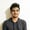# How to Code a Random Color Generator in JavaScriptColors are a crucial part of design, both online and offline. Almost all elements on a webpage have some color applied to them. There are entire websites built around creating gradients and color palettes either randomly or from a source color.

In this tutorial, I'll show you how to generate a random color in JavaScript. Maybe you will find the random color generator useful when developing a website that lets users create stylish posters or form elements!

## Understanding the Basic Concept

Colors can be represented in a lot of formats like RGB, Hexadecimal, or HSL notation. We will generate a random color in these three notations. Generating a random color is simply a matter of generating random numbers.

Luckily, JavaScript does have a Math.random() function that we can use to generate those random numbers. It will give you a random floating-point pseudo-random number that will be greater than or equal to 0 and less than 1. The distribution is pretty much uniform. This is good enough for our use case.

We will be generating random numbers between specific ranges like 0 to 100 or 0 to 255. Basically, the minimum value we want to get from our random number generator is 0, and the maximum value can be whatever we want, like 100 or 255.

Multiplying any number by 0 will give us 0, and multiplying it by 1 will give us the same number back. So multiplying our desired maximum value—for example 255—by anything between 0 and 1 will give us a number between 0 and 255. However, since Math.random() never gives us 1 exactly, we will have to use some other trick to get 255. This can be done by internally increasing the maximum limit by 1.

Here is the implementation of this logic as a function in JavaScript.

Multiplying a number by some random fraction doesn't always result in integer values. Therefore, we use the Math.floor() function to get rid of the decimal part from our numbers.

## Generating Random Colors

We will now write the code to create random colors in RGB, hex, and HSL notation.

### Generating an RGB Color Value

This requires us to create three random integers between 0 and 255. These values will represent the red, green, and blue components of our colors. After that, we simply need to do some basic string concatenation. Here is our function for generating random RGB color values in JavaScript:

### Generating a Hex Color Value

Just like the previous example, we will begin by generating a random number between 0 and 255. After that, we will convert those numbers to the corresponding hexadecimal values. A simple string concatenation in the end will give us our random color in hex format.

You might have noticed that we are also using the padStart() function. This is because the random number that we generate will sometimes be represented by a single digit when converted to hexadecimal notation. However, a valid color in this format needs to have 3 or 6 characters. Padding with a 0 at the beginning will give us a total character sequence length of 6.

### Generating an HSL Color Value

Color in HSL notation also consists of three numbers. The first one is an angular degree that determines the hue of the color, the second value is a percentage that determines the saturation of the color, and the third value is used to specify the lightness of the color as a percentage. Hue can range from 0 to 360, while the percentages will vary from 0 to 100.

## Random Color Generator

Here's a CodePen example of the random color generating functions in action!

## Final Thoughts

This quick tip showed you how to generate random colors in JavaScript in three common formats: RGB, hex, and HSL. If you want to have transparent colors, you can use the same technique to randomize the opacity of your colors.